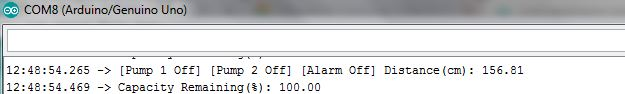# Limit serial print variable value to a specific range

This is only a minor annoyance and does not actually affect the running of my program in anyway, it is more a curiosity of how i would solve it if it was critical to the program operation for future reference.

The issue i have is my program has a variable Capacity Remaining % which i print to the terminal.

``````  // Return Value Serial Monitor
Serial.print("Distance: ");
Serial.print(DISTANCE);
delay(200); // 0.2s Update Cycle
Serial.print("Capacity Remaining: ");
Serial.print(PERCENT);
delay(200); // 0.2s Update Cycle
``````

This value is calculated with the following code snippets:

``````// User Defined Variables

int TANK_DEPTH = 100; // Sump Depth Setting (cm)
``````
``````// Variables For UltraSonic Sensor Calculation
float VELOCITY = 0.034;          // Speed of Sound 340 m/s in cm/us
long  DURATION;                  // Time Trig -> Echo
float DISTANCE;                  // Distance (cm)
float PERCENT;                   // Sump Capacity Percentage (%)
float VAL_A = 100/TANK_DEPTH ;   // Used in Percentage Calculation
``````
``````  // Read Echo Pulse Travel Time
DURATION = pulseIn(US_ECHO_PIN, HIGH);

// Calculate Distance Measurement:
// Speed = Time x Velocity
// Distance = Time x Velocity / 2
DISTANCE = DURATION*VELOCITY/2;

// Calculate Capacity Percentage
PERCENT = VAL_A*DISTANCE;
``````

When the sensor reads a value over the defined Tank Depth(=100) i.e 110cm it reads 110% how would i make the code so that it ignores any value over 100% and just displays 100% ???

As i say this wouldn't occur in this application as it would never read over 100% and has only occurred while i am testing i am just curious.

``````if(PERCENT > 100)
{
PERCENT = 100;
}
``````

Do you mean something like that?

groundFungus:

``````if(PERCENT > 100)
``````

{
PERCENT = 100;
}

``````

Do you mean something like that?
``````

That will do the trick why do i always think the solution wont be so obviously simpleThanks

Because things that seemingly should be simple in C++ are so often not?

That's not even the simplest solution. You could also use constrain(), which under the hood probably does the same:

``````PERCENT = constrain(PERCENT, 0, 100);
``````

Another thing: all capital variable names are normally used to distinguish constants such as your VELOCITY (which presumably never changes). Those variables normally then also have the const keyword added to their declaration:

``````const float VELOCITY = 0.034;
``````

Now if you still try to change the value somewhere in the code, the compiler throws an error.

Thanks for the additional info i wasn't aware of that, i have used capitals for all of my variables its a habit didnt know it was a bad one.

I have another question in regards to printing on the serial terminal, is it possible to specify a new line for each Serial.print ?

I have the following code and it all prints on the same line, again it isnt a major issue just curious if there is a solution?

``````   // Pump('s) Off
digitalWrite(PIN_PUMP_1_ACTIVE, PUMP_OFF);
digitalWrite(PIN_PUMP_2_ACTIVE, PUMP_OFF);
Serial.print("[Pump 1 Off] ");
Serial.print("[Pump 2 Off] ");

// Alarm Warning Disabled
digitalWrite(PIN_ALARM_1, ALARM_OFF);
Serial.print("[Alarm Off] ");
````````````// Pump('s) Off
digitalWrite(PIN_PUMP_1_ACTIVE, PUMP_OFF);
digitalWrite(PIN_PUMP_2_ACTIVE, PUMP_OFF);
Serial.println("[Pump 1 Off] ");  // println() function
Serial.println("[Pump 2 Off] ");

// Alarm Warning Disabled
digitalWrite(PIN_ALARM_1, ALARM_OFF);
Serial.println([Alarm Off] ");
``````

An then there is the line feed character that you can use instead of println(). The println() function automatically sends a line feed and carriage return after the print data. Most of the time sending a line feed does the same as println().

Some ways to print to the next line without println(). Six lines without println:

``````void setup()
{
Serial.begin(115200);
Serial.print("line one");  // reference line
Serial.print('\n');  // send just line feed
Serial.print("line two");
Serial.print("\nline three");  // insert a line feed into a string
Serial.write(10);  // send DEC value of line feed
Serial.print("line four");
Serial.write(0x0a); // send HEX value of line feed
Serial.print("line five");
Serial.print("\r\n");  // the same as println()
Serial.print("line six");
}

void loop()
{

}
``````